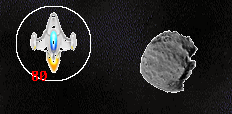### How to Draw a circle in CIn the asteroids game, when you press the s button to put up a shield, it draws a circle.  I must confess, I didn’t know how to draw a cuircle so looked it up and found an example on StackOverflow. You can use code from StackOverflow, licensed under a MIT license.  I include the link to StackOverflow in the game code (in the chapter 48 zip file) in a comment.

Here’s the code in the game.

``````void DrawCircle(SDL_Renderer *Renderer, int _x, int _y, int radius)
{
int x = radius - 1;
int y = 0;
int tx = 1;
int ty = 1;
int err = tx - (radius << 1); // shifting bits left by 1 effectively
// doubles the value. == tx - diameter
while (x >= y)
{
//  Each of the following renders an octant (1/8th) of the circle
SDL_RenderDrawPoint(Renderer, _x + x, _y - y);
SDL_RenderDrawPoint(Renderer, _x + x, _y + y);
SDL_RenderDrawPoint(Renderer, _x - x, _y - y);
SDL_RenderDrawPoint(Renderer, _x - x, _y + y);
SDL_RenderDrawPoint(Renderer, _x + y, _y - x);
SDL_RenderDrawPoint(Renderer, _x + y, _y + x);
SDL_RenderDrawPoint(Renderer, _x - y, _y - x);
SDL_RenderDrawPoint(Renderer, _x - y, _y + x);

if (err <= 0)
{
y++;
err += ty;
ty += 2;
}
else
{
x--;
tx += 2;
err += tx - (radius << 1);
}
}
}``````

it’s as simple as that! To make it more interesting, it is called each frame with the shield throbbing  by increasing  the radius from 38 to 46 pixels by 2 then restarting at 38 again. Here’s the code for that. Note that when the shield energy is below 10, it no longer works.

``````void DisplayShield(SDL_Rect * target) {
if (shieldFlag && shieldStrength >10) {
SDL_SetRenderDrawColor(renderer, 0xff, 0xff, 0xff, 0xff);
DrawCircle(renderer, target->x + (SHIPWIDTH/2), target->y + (SHIPHEIGHT/2), shieldRadius);